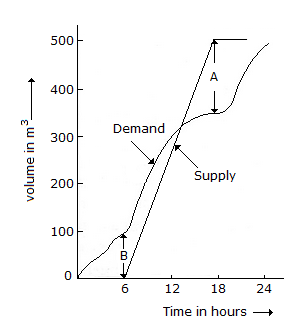# Civil Engineering - UPSC Civil Service Exam Questions

11.

What is the BOD5 at 20°C of a waste that yields an oxygen consumption of 2 mg/l from a 0.5% diluted sample ?

 A. 50 mg/l B. 400 mg/l C. 200 mgl D. 250 mgl

Explanation:

No answer description available for this question. Let us discuss.

12.

The given figure shows roughly, the daily mass curves of supply to and demand from an elevated service reservoir (ESR). The minimum required capacity of the reservoir is given byA. (A - B) B. (A + B) C. A x B D. larger of A or B

Explanation:

No answer description available for this question. Let us discuss.

13.

Stresses obtained from Boussinesq's theory are considered reasonably satisfactory in foundation engineering because:

 A. they represent stress distribution in homogeneous soil below loaded area B. they account for anisotropy of soil properly C. they give due regard to plastic behaviour of soils, particularly of settlement analysis D. they consider elastic soil medium, and the intensity of allowable stresses below foundations in most cases are quit small and justify elastic solutions

Explanation:

No answer description available for this question. Let us discuss.

14.

A septic tank of 7 m3 in volume serves for 5 people. If the rate of accumulation of sludge is 70 litres per capita per year and sludge is removed when it occupies 50% of its volume, what is the cleaning interval of septic tank ?

 A. 3 years B. 5 years C. 7.5 years D. 10 years

Explanation:

No answer description available for this question. Let us discuss.

15.

The load carrying capacity of an individual friction pile is 200 kN. What is the total load carrying capacity of a group of 9 such piles with group efficiency factor of 0.8 ?

 A. 1800 kN B. 1640 kN C. 1440 kN D. 900 kN